Solenoidal Field

A solenoidal Vector Field satisfies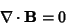(1)

for every Vector, where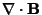is the Divergence. If this condition is satisfied, there exists a vector, known as the Vector Potential, such that(2)

whereis the Curl. This follows from the vector identity(3)

Ifis an Irrotational Field, then(4)

is solenoidal. If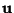andare irrotational, then(5)

is solenoidal. The quantity(6)

where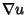is the Gradient, is always solenoidal. For a function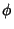satisfying Laplace's Equation(7)

it follows thatis solenoidal (and also Irrotational).